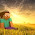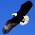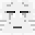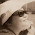## Wednesday, 27 February 2013

### Algebra... Which is larger? (II)

Jennifer says, "5x2  is always smaller than  (-5x)2. "

This question is compulsory.

1.no, i do not agree.

because in " (-5x)^2 " the magnitude and the negative sign are both in the bracket, there fore you would have to square the whole thing, inclusive of the negative sign. a negative x a negative gives you a positive and therefore when you square a negative number, it becomes a positive, so you can just ignore the negative sign in " (-5x)^2 " and calculate 5x^2 to get your ans. since " (-5x)^2 " is the same as 5x^2,the statement "5x2 is always smaller than (-5x)2 " is wrong.

2.No.
Since both x has the same value and when two negative number multiplied together will be a positive number,the two sums above will be the same as (5x)(5x) is equal to (-5x)(-5x).

3.No. Both equations when done would give the same answer.

4.No.If you work out both the equations,the answer will be the same so Jane is wrong.

5.No. The answer will be the same as the second equation has a bracket so the negative sign will be included in the multiplication and hence the answer will be the same.

6.no the answer will become the same when both equation are evaluated

7.No. When X is an any no. no matter what the answer become the same. (5X)*(5X) = (-5X)*(-5X) Since negative and negative gives us positive

8.No, indeed not. The answer will be the same.

9.I do not agree because the number does not change at all except for the negative sign which will be turned into a positive sign when it is squared. Hence it does not change.

10.No I do not agree. Because since -ve * -ve = +ve, (-5x)^2 can be simplified to 5x^2. Therefore the answers would be the same for both equations

11.I do not agree. Both values have the same answer. Positive*positive=positive and Negative*negative=positive, hence there is no difference.

12.I do not agree as once you worked them out, the answers would be the same and there's no difference in the answers at all.

13.I do not agree as 5x^2 is equal to (5x)(5x) . (-5)^2 is (-5)(-5) will be same as (5)(5) because the two negative signs are changed to positive.

14.Let's expand it:
5x^2 = 5 * x^2
(-5x)^2= (-5)(-5)(x)(x)
=25x^2
SO when we compare (-5x)^2 and 5x^2,
(-5x)^2 is greater.
So i don't agree with her.

15.This comment has been removed by the author.

16.The answers will be the same as (-)x(-)=(+) so i dont agree with Jennifer

17.The answers are the same
5x^2 = 5 * x * x.
(-5x)^2 = 5*x*x(The negative signs will be cancelled.)

18.Looks like almost all disagree that 5x^2 is always smaller than (-5x)^2.
Some claim that they are the same - ok, when you choose one 'special' value to test.
Does anyone else have a different view?